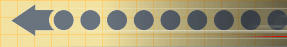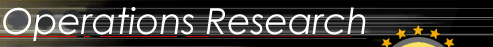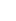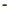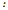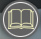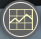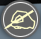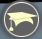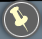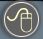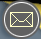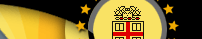An introduction to the basic mathematical ideas and computational methods of optimizing allocation of effort or resources, with or without constraints.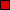Linear programmingSimplex MethodNetwork modelsDynamic programmingInteger programmingTwo-person, zero-sum gamesMarkov chainsQueueing theory    This course is an introduction to the basic mathematical ideas and computational methods of optimization including linear programming, the theory of optimal decision making with a linear objective function and under linear constrains on resources. Applications include decision theory in economics, transportation theory, optimal assignments, production and operations scheduling, network modeling and flows, dynamic programming, and integer programming. A student is expected to learn how to use a software package (such as Excel solver, LINGO/LINDO, MPL, CPLEX, etc.). Prerequisite: An intorduction to matrix calculations.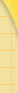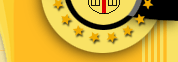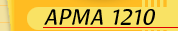ReadingSyllabusGradingExams & SolutionsHomeworkUtilities/softwareapplicationsContactComputingMain WebsiteAPMA 0330APMA 0340APMA 1210APMA 2140